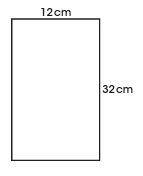# Third Term Examination Mathematics SS 1 – Exam Questions

### SECTION A

1. Find x, if x² – 8x + 15 = 0.

(a) 5 , 3

(b) – 5 , – 3

(c) – 5 , 3

(d) 5 , – 3

2. U means __________ in set theory.

(a) unity

(b) union

(c) universal

(d) unicode

3. __________ is the Area of a trapezium.

(a) ½bh

(b) ½(a + b)h

(c) b x h

(d) ¾(a + b)h

4. Reduce (30a²b)/(20ab²) to the lowest term.

(a) 3a/2b

(b) 3ab/2a

(c) 3a²b/2ab²

(d) 15ab/10b

5. {1, 2, 3, 4, 5, _, _, _, _} is __________ set.

(a) null

(b) subset

(c) infinite

(d) universal

6. Write 24.6 x 10^-5 in a standard form.

(a) 24.6 x 10^-6

(b) 25.0 x 10^-5

(c) 2.46 x 10^-4

(d) 2.46 x 10^-7

7. All are polygons except __________.

(a) triangle

(b) rectangle

(c) sphere

(d) pentagon

8. If 4m + 6m + 10m – 10m = 50, solve for m

(a) 3/5

(b) 5/3

(c) 5

(d) 3

9. If U = {L, O, N, D, O, N} and S = {O, N, D, O}, then S’ = __________.

(a) Ø

(b) {L, O, N, D}

(c) {L, N}

(d) {L}

10. (d – 5) (3d – 2) = 0,  d = __________ , __________

(a) 5 , 2/3

(b) 5 , 3/2

(c) 5 , 3

(d) 5 , 2

11. A  ⊆ B means A __________ set B

(a) element of

(b) subset of

(c) contains

(d) union

12. ∩ means __________.

(a) union

(b) entity

(c) intersection

(d) contain

13. The underlined word in SOHCAHTOA means __________.

(a) SinӨ = opp/hyp

14. All are negative in 2nd quadrant except __________.

(a) sine

(b) cosine

(c) tangent

(d) Acts

15. Another name for ideograph is __________.

(a) histogram

(b) pictogram

(c) pie chart

(d) bar chart

16. Theorem __________ states that sum of the interior angles of any n-sided convex polygon is (2n – 4) right angle.

(a) 1

(b) 2

(c) 3

(d) 4

17. __________ is the most occurring figure in a frequency table.

(a) Mean

(b) Median

(c) Mode

(d) Range

18. The mid-point of class interval is called class __________.

(a) mid – value

(b) limit

(c) boundaries

(d) width

19. __________ means ∑(F)/F.

(a) Mean

(b) Median

(c) Mode

(d) Range

20. All are 3-dimensional shapes except __________.

(a) cube

(b) cuboid

(c) sphere

(d) parallelogram

### SECTION B

QUESTION 1

The shoe sizes of a group of 24 children are –

### 8  5  4  6  5  5  6  7  8  8  6  7

Using the data above,

A. Re-arrange the data in an ascending order

B. Prepare a frequency table.

QUESTION 2

A. Construct angle 90°, 45° and 60°.

B. Find the mean of the data in question 1 above.

QUESTION 3

A. Find the area and perimeter of the shape in cmB. Using the class interval 11 – 20, 21 – 30, __________.

I. Construct a frequency table.

II. What is class boundaries of figure 2?

The following is the record of marks of 40 candidates in an examination;

### 22  41  27  51  63  47  19  35  39  76

QUESTION 4

A. Construct a rectangle with length 12cm and breadth 16cm

B. State the area and perimeter of:

I. Rectangle

II. Parallelogram

III. Square

QUESTION 5

A. Find the area of the sector with angle 60° and radius 7cm.

B. State the 1st 3 theorems of deductive proof.

One Comment
1.THANKS FOR USING CLASSROOMNOTE!!!